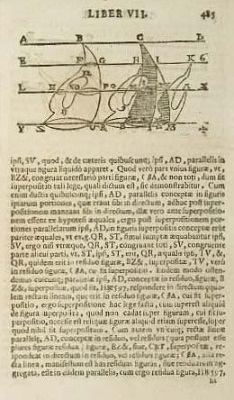## The CSHPM/SCHPM Logo

In the seventeenth century, Italian mathematician Bonaventura Cavalieri published a treatise on the use of "indivisibles" to calculate areas and volumes: Geometria Indivisibilibus Continuorum Nova quadam ratione promota. At the start of the seventh book in the work, Cavalieri states the following theorem:

If between the same parallels any two plane figures are constructed, and if in them, any straight lines being drawn equidistant from the parallels, the included portions of any one of these lines are equal, the plane figures are also equal to one another; and, if between the same parallel planes any solid figures are constructed, and if in them, any planes being drawn equidistant from the parallel planes, the included plane figures out of any one of the planes so drawn are equal, the solid figures are likewise equal to one another.

Now known as Cavalieri's Principle, the theorem could be rephrased as saying that two solid regions sharing equal area cross-sections at every height must have the same volume, and that two planar regions sharing equal length cross-sections at every height must have the same area.

Cavalieri's work can be viewed as one step in the evolution and development of the integral calculus. The image used in the CSHPM/SCHPM logo appears as part of Cavaleri's justification of the theorem (see page at left).

[Note: the above English translation of Cavalieri's theorem comes from the article "Cavalieri's Theorem in His Own Words," by G. W. Evans, The American Mathematical Monthly, Vol. 24 No. 10, Dec. 1917, 447-451. The image of the full page to the left comes from the second edition of Geometria Indivisibilibus Continuorum, published in Bologna in 1653.]